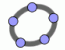ProfessorBurke.org Where Mathematics, Education and Technology Meet Interactive Manipulatives - Powered by GeoGebraStatistics Manipulatives (v3.0) Algebra Manipulatives (v2.0) Vector Manipulatives (v2.0) Conic Section Manipulatives (v2.0) Parametric Equations Manipulatives (v2.0) Arithmetic Manipulatives (v3.0) Trigonometry Manipulatives (v2.0) Calculus Manipulatives (v2.0) Generalized Parabolas (v2.0) Contact Me                                                       © 2007-2018 John Burke